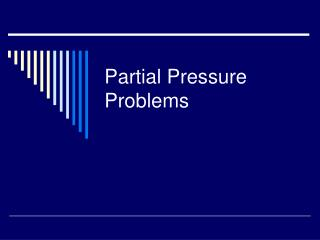Download PresentationPartial Pressure Problems

# Partial Pressure Problems - PowerPoint PPT PresentationDownload Presentation## Partial Pressure Problems

- - - - - - - - - - - - - - - - - - - - - - - - - - - E N D - - - - - - - - - - - - - - - - - - - - - - - - - - -
##### Presentation Transcript

1. Partial Pressure Problems

2. Vocabulary Used in Partial Pressure Problems • Partial Pressure- The pressure of each gas in a mixture. • Dalton’s Law- The total pressure of a gas mixture is the sum of the partial pressures of the component gases. • Pt = P1 + P2 + P3 +… • Mole Fraction- the mole of the gas being used divided by the total moles in the mixture.

3. Explaining Further Given an ideal gas mixture of nitrogen (N2), hydrogen (H2), and ammonia (NH3) the total pressure would be the sum of all three of the gases’ partial pressure, as stated in Dalton’s Law.

4. Basic Partial Pressure Problems A container holds three gases: oxygen, carbon dioxide, and helium. The partial pressures of the three gases are 2.00 atm, 3.00 atm, and 4.00 atm, respectively. What is the total pressure inside the container? This one is easy, simply add the pressures together. 2.00 + 3.00 + 4.00 = 9.00 atm. So the total pressure is 9.00 atm.

5. Partial Pressure problems using water displacement. Many of the partial pressure problems done in high school use water displacement, and with water displacement comes water vapor pressure. If only one gas is collected, the only two gases present are the collected gas and water vapor. So the equation looks like this. Water vapor pressure depends only on the surrounding temperature. There is a water vapor pressure chart here.

6. Water Displacement Problems Here is an easy partial pressure problem using water displacement: If 60.0 L of nitrogen is collected over water at 40.0 °C when the atmospheric pressure is 760.0 mm Hg, what is the partial pressure of the nitrogen? Given: 760.0 mm Hg is the total pressure. The water vapor pressure at 40.0°C is 55.3 mm Hg. This is found on the chart hyperlinked on the previous page. The 60.0 L of nitrogen is not needed in this problem. Now all you do is subtract the water vapor pressure from the total pressure to get 704.7 mm Hg. So the partial pressure of nitrogen is 704.7 mm Hg.

7. Mole Fractions Mole fractions can also be used in partial pressure problems. Here is an easy sample problem using mole fractions: A tank contains 160.0 grams of oxygen and 40.00 grams of helium, what is the mole fraction of the two gases? There are 5.00 moles of oxygen and 10.0 moles of helium, thus there are 15.0 moles of gas in the tank. To find the mole fraction of the gases divide the moles of each gas by the total moles in the tank. Since 5 ÷ 20 is 0.333, the mole fraction of oxygen gas is 0.333. You can think of the mole fraction of He as being ether 10/15 = 0.667 or as being 1-0.333, also 0.667.

8. Mole Fractions Continued Mole fractions are very useful because they also tell you the partial pressure of the gas. Let’s go back to the previous problem. Suppose there is 100.0 kPa of pressure in the tank. You simply multiply the mole fraction by the total pressure. So take the mole fraction of oxygen, 0.333, and multiply it by 100.0 kPa. So the partial pressure of oxygen is 33.3 kPa, or 33.3% of the total pressure.

9. One Last Problem A tank contains 480.0 grams of oxygen and 80.00 grams of helium at a total pressure of 7.00 atmospheres. Calculate the following. • How many moles of O2 are in the tank? • How many moles of He are in the tank? • Total moles of gas in tank. • Mole fraction of O2. • Mole fraction of He. • Partial pressure of O2. • Partial pressure of He.

10. Answers to Previous Problem a) Simply convert grams of Oxygen to moles of O2. Look up the molar mass of oxygen, it is 16g. So there are 30.0 mol of O2 in the tank. b) Do the same as a). So there are 20.0 mol of helium in the tank.

11. Answers Continued c)Simply add the moles of the two gases together. 15.0 moles of 02 + 20.0 moles of He = 35.0 moles of gas in the tank. d) To find the mole fraction divide the moles of the gas by the total moles of gas in the mixture. 15.0 mol of 02÷ 35.0 mol of total gas = 0. 429. So the mole fraction of 02 is 0.429. e) Do the same as in d) but for Helium. 20.0 mol of He ÷ 35.0 mol of total gas = 0.571. So the mole fraction of Helium is 0.571. f) To find the partial pressure of the oxygen gas multiply the mole fraction by the total pressure. 0.429 x 7 atm = 3.00 atm. So the partial pressure of the oxygen gas in the tank is 3.00 atm. g) Do the same as in f) but for the helium gas. 0.571 x 7 atm = 4.00 atm. So the partial pressure of the helium gas in the tank is 4.00 atm.